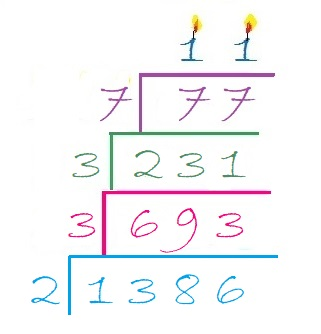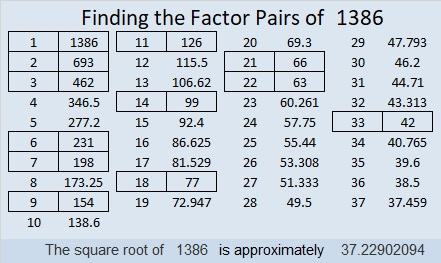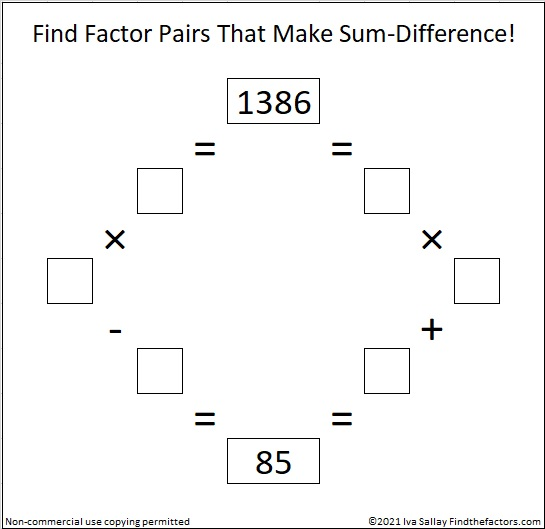# 1386 What You Need to Know About the Multiplication Game

Contents

I recently wrote about Hooda Math’s Multiplication Game. There’s a couple of things I didn’t tell you in that post.

First of all, you don’t have to use a computer to play the game. (However, using one the first time you play will help you understand how to play). You can print a game board to play. I’ve created a game board below that you could use. Each player can use different items such as beads, pennies, nickels, and dimes as markers to mark the factors used and to claim the resulting products on the game board.The second thing you should know is that getting four squares in a row, horizontally, vertically or diagonally is NOT equally likely every place on the board. If one particular number is all you need to get a win, you are less likely to get that number if it only has one factor (like the numbers marked in yellow have). As far as this game is concerned, the products have the number of factors that I’ve indicated, even though in reality most of them have more than that.

You can’t win unless your opponent gives you one of the factors you need to claim that winning space. If 4 of the 9 possible factors will get it for you, the odds are much better your opponent will give you what you need than if only 1 of the 9 possible factors will do it.

If you know which numbers have four possible factors, you may have an advantage over someone who thinks this game is really just a variation of tic-tac-toe. Of course, those products with four factors could also make you more likely to get blocked as well! And if you use my colorful game board, your opponent will know just as much as you do about how many ways they can get each square.

### 1386 Factor Cake:

Since the biggest prime factor of 1386 is 11, it makes an especially festive factor cake!### Factors of 1386:

Now I’ll share some information about the number 1386:

• 1386 is a composite number.
• Prime factorization: 1386 = 2 × 3 × 3 × 7 × 11, which can be written 1386 = 2 × 3² × 7 × 11
• 1386 has at least one exponent greater than 1 in its prime factorization so √1386 can be simplified. Taking the factor pair from the factor pair table below with the largest square number factor, we get √1386 = (√9)(√154) = 3√154
• The exponents in the prime factorization are 1, 2, 1, and 1. Adding one to each exponent and multiplying we get (1 + 1)(2 + 1)(1 + 1)(1 + 1) = 2 × 3 × 2 × 2 = 24. Therefore 1386 has exactly 24 factors.
• The factors of 1386 are outlined with their factor pair partners in the graphic below.### Sum-Difference Puzzle:

1386 has twelve factor pairs. One of the factor pairs adds up to 85, and a different one subtracts to 85. If you can identify those factor pairs, then you can solve this puzzle!### One More Fact about the Number 1386:

OEIS.org also noted that 1 + 3⁴ + 8 + 6⁴ = 1386.

This site uses Akismet to reduce spam. Learn how your comment data is processed.